## Example Questions

### Example Question #2 : Decimals With Fractions

A tub of food containspounds of vegetables,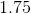pounds of lard, andpounds of sausage.  What is its total weight as an improper fraction?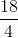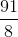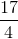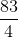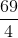Explanation:

To begin with, it is easiest just to add these decimals together using your calculator: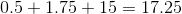Now, this is the same thing as: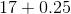We can rewrite this: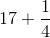To find this, you need to give the two numbers a common denominator: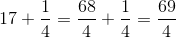### Example Question #2 : How To Find The Fractional Equivalent Of A Decimal

What is the fractional equivalent of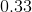?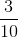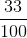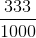Explanation:

In decimal formis said 33 hundredths.

This is equal to.

This fraction cannot be reduced any further therefore it is in its final answer form.

### Example Question #1 : How To Find The Fractional Equivalent Of A Decimal

Choose the answer which best converts the following decimal into a fraction, ensure that you simplify your answer: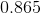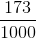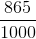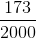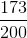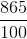Explanation:

To convert a decimal into a fraction, simply put the digits of the decimal in the numerator of a fraction, then one followed by a number of zeroes equal to the number of digits in the decimal as your denomator.  In this case:Then, you can simplify, as both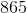and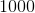are divisible by:### Example Question #2 : How To Find The Fractional Equivalent Of A Decimal

Simplify the following expression: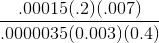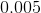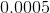Explanation:

It can help to begin by writing the expression using scientific notation: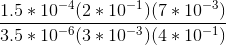Now remove common divisors from the numerator and denominator: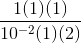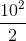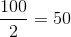Tired of practice problems?

Try live online GRE Math prep today.